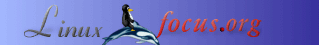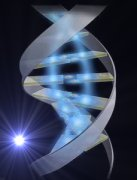<--  | Map  | Index  | SearchThis document is available in: English  Castellano  Francaisby Carlos Andrés Pérez About the author: Carlos Andrés Pérez is specialist in Molecular Simulation, Candidate to Doctor in Biotechnology. Technical advisor of the Grupo de Investigación en Educación Virtual (GIEV) - Research Group in Virtual Learning. Address: Universidad Santiago de Cali, Calle 5ª carrera 62 Campus Pampalinda, Cali – Colombia. Content:

 PDF

## Computer Assisted Simulation of DNA using Linux and PerlAbstract:

This article shows a way to generate “n” DNA sequences with “s” nucleotides, in a random way using Perl programs. In contrast with other programs where DNA sequences are processed as text chains, this article proposes to work with them as arrays. Even with the random generation of the sequences, when we do the statistical analysis of the media fraction of the identical nucleotides that are placed in the same comparative position, we obtain values similar to 0.25.

_________________ _________________ _________________

## Computer simulation

The scientific research is focused on the study and understanding of processes in nature. The research has been done using experimental observation and theoretical modeling, which, for a long time has been dedicated to exposition and development of equations. But many of these equations can't be solved analytically or numerically because of technical aspects or the impossibility of representing nature.

The ever growing development of computing sciences leaves us to reach the natural phenomenons through software programs that can replace the mathematical equations for describing natural systems. These programs ease the introduction of random variables that ease the reproduction of biological and physical processes in the computer, finding deterministic aspects in the simulations, that can be translated after wards into laws.

## Why perl?

Perl (Practical Extraction and Report Language), is an structured language that handles data of scalar, list, array, hashes and files types. It is already versatile in its applicability, because it is easy to generate functions that can be evaluated with any data type, besides to its capability of integration in databases, dynamic websites and operating systems like Linux, assisting the end user with common tasks.

Perl is very attractive as analytic tool because its syntax allows us to work with very similar structures to Mathematica, a powerful symbolic language ( http://www.xahlee.org/PerlMathematica_dir/perlMathematica.html ), and its modules can be integrate with C, GTK, GGI, GGL libraries, adding the capabilities of integrating programs in a single language.

There are several programs with a big advantage in the data management, structured in a mathematical way of programming. But these are commercial software packages and this limits its reproduction and improvement. At the same time they are very restrictive when communicating with other languages.

Perl doesn't limit the data size, it depends on the memory capabilities and the recursive resources. The number of hash tables used in associative arrays isn't also limited.

In this article every DNA chain has been represented as a vector. We can use the PDL module (Perl Data Language, (http://pdl.sourceforge.net/WWW-old/index_es.html ), which is oriented to the numeric processing of data through n-dimensional matrices, but we have trouble defining each vector element as a nucleotide, because the “pdl” function only handles numeric orders. For that reason we proceed to use the Perl basic functions for handling arrays, anyway this doesn't limits the possibility of representing each nucleotide as a number.

## Random sequences

The program, let us generate “n” DNA chains composed of “s” nucleotides. Each chain has the same length and its components are nucleotides randomly chosen. The program converts the scalar list passed as parameter by the Dumper function in a Perl chain that describes the data structure, so we can visualize each vector.

```#!/usr/bin/perl
# Use the module Data::Dumper
use Data::Dumper;
# In \$var1 the sequences number is stored, in \$var2 the chain length.
print "Enter the number of random sequences to be generated\n";
\$var1 = <STDIN>;
print "Enter the number of nucleotides per sequence\n";
\$var2 = <STDIN>;
# In aleatorio we define the array @ns that contains the nucleotides,
# \$lon is the local variable that stores the number of nucleotides
# \$col is the local variable that stores the number of
# sequences
sub aleatorio {
local @ns = (a,g,c,t);
local \$lon = \$_;
local \$col = \$_;
# We define an empty array  @a to store the vectors.
@a = ();
# The variables that indicate the vectors and its componnent?s positions.
local \$i = 0;
local \$u = 0;
while (\$u <= \$col - 1 && \$i <= \$lon - 1) {
# \$result is the variable that stores the random election of every of the
# nucleotides.
\$result = @ns[int(rand(4))];
# Add nucleotides and store the chains that contains them.
\$a[\$u][\$i] = \$result;
\$i = \$i + 1;
if (\$i == \$lon ) {
\$u = \$u + 1;
\$i = 0;
}
}
return @a;
}
#Show in the creen every vector and its components.
print Dumper(&aleatorio(\$var1,\$var2));
# Define positions and inicial vectors used to compare
# if they have identical nucleotides in the same positions.
\$k = 0;
\$count = 0;
\$s1 = 0;
\$s2 = 1;
while (\$s1 <= \$col - 2  &&  \$s2 <= \$col - 1 && \$k <= \$lon - 1 ) {
# If they are identical \$count increases in 1.
if (\$a[\$s1][\$k] eq \$a[\$s2][\$k]) {
\$count = \$count + 1;
}
# \$k indicates nucleotides in the vector, \$s1 and \$s2 are the vectors being compared.
# If \$k  value is the same at the nucleotides number is a flag
# that the comparation has finished.
\$k = \$k + 1;
if(\$k == \$lon ) {
\$k = 0;
\$s2 = \$s2 +1;
}
# If \$s2 is the same than \$col, we know that one of the vectors has been
# compared with the others.
if (\$s2 == \$col ) {
\$k = 0;
\$s1 = \$s1 + 1;
\$s2 = \$s1 + 1 ;
}
}
# We determine \$pvalue
# which shows the number of comparisons.
for (\$p = \$col - 1, \$r = \$col -2; \$r >= 0 ; \$r--){
\$p+= \$r;
}
# The results are shown.
print "The number of identical nucleotides is: \$count\n";
print "The number of comparisons is: \$p\n";
\$y = \$count/\$p;
# In \$cor we store the media fraction value of
# identical nucleotides in the same position
#during the comparison.
\$cor = \$y/\$lon;
print "The media fraction of nucleotides appearing in the same position is: \$cor";
```

Estimating the \$cor value for 10 sequences of 30, 50, 70 and 90 nucleotides we get the following results: 0.2340, 0.26, 0.26031, 0.2661.

For 40 sequences of 100, 200, 300 and 500 nucleotides we get the following values of \$cor: 0.2507, 0.2482, 0.2480, 0.2489.

According to that we conclude that for “n” number of DNA sequences with “s” nucleotides and randomly generated, show a media fraction of identical nucleotides that are in the same position around 0.25.

## Bibliography

 talkback page

 <--, LF Home Go to the index of this issue

Webpages maintained by the LinuxFocus Editor team